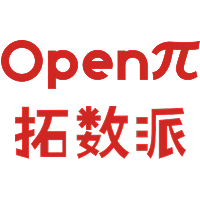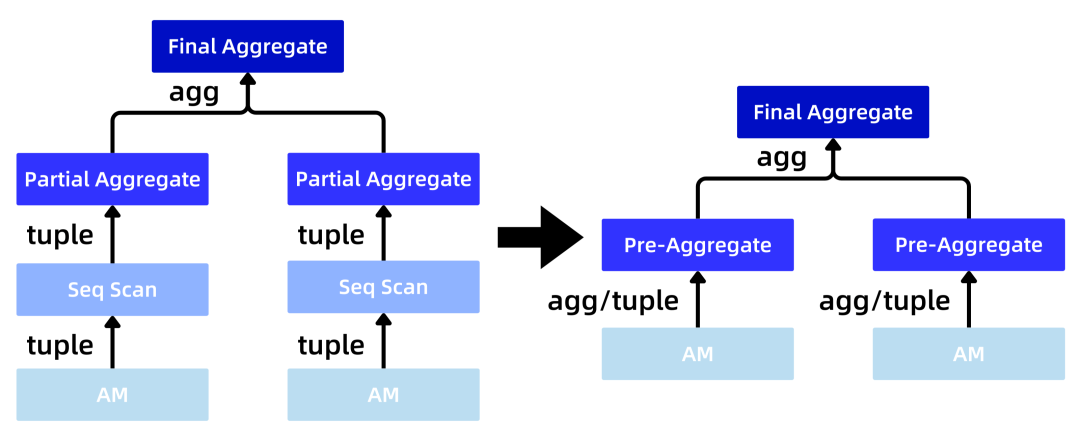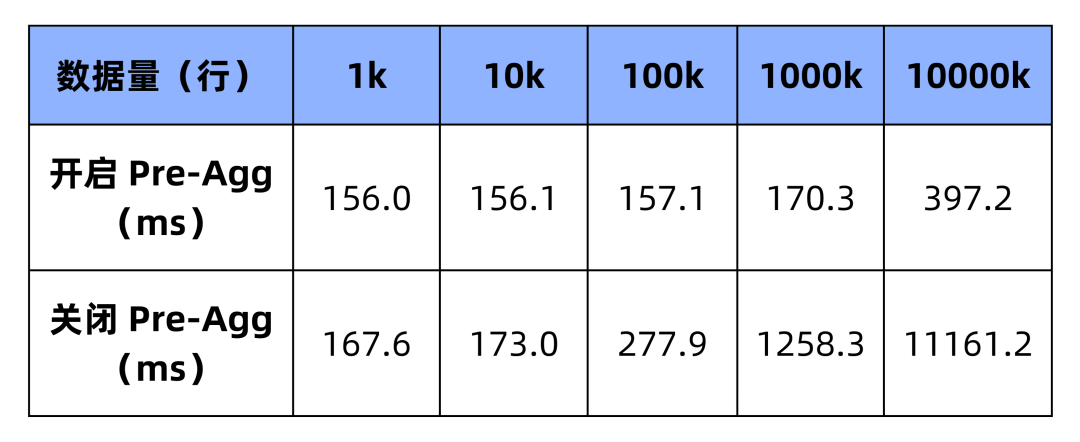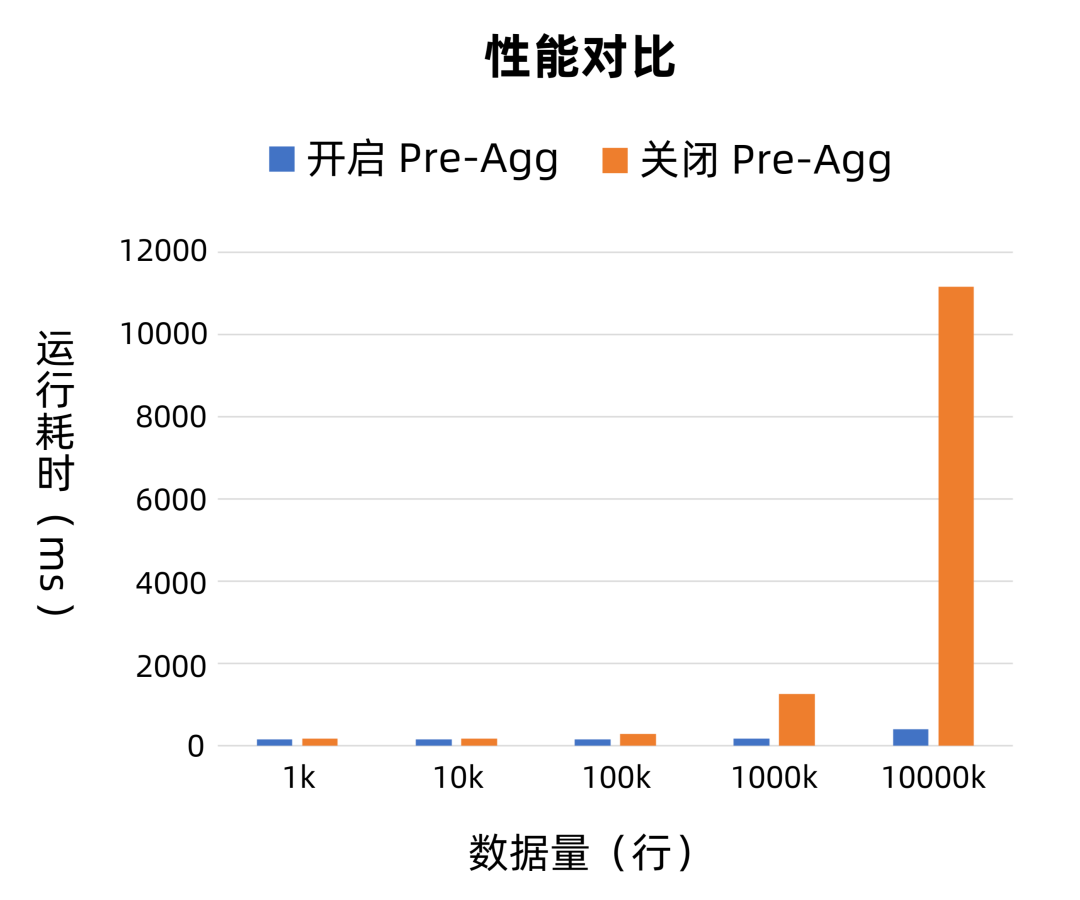# 领先一步，效率翻倍：PieCloudDB Database 预聚集特性让查询速度飞起来！2023-10-19

PieCloudDB 的设计理念源于对用户使用体验和查询效率的深度理解。在实现存算分离的同时，PieCloudDB 专门设计和打造了全新的存储引擎「简墨」等模块。针对云场景和分析型场景，PieCloudDB 还设计了高效的预聚集（Pre-Aggregate）特性。本文将详细介绍 PieCloudDB 如何运用预聚集技术优化数据处理流程，改善用户体验。

PieCloudDB 实现了一个重要创新功能：预聚集（Pre-Aggregate）。该功能通过 PieCloudDB 的全新的存储引擎「简墨」（JANM），在数据插入时即时计算数据列的 Aggregate 信息，并将其预先保存以供后续使用。这种方法摒弃了在查询时进行复杂计算的传统方式，从而大大提升了查询速度。此外，由于聚合数据保存在文件中，可以实现快速访问并直接应用于查询。

PieCloudDB 会根据用户的查询自动生成带有 Pre-Aggregate 的计划，使得查询过程尽可能地快速且准确。当需要聚合数据时，系统会检查预存储的聚合值，并直接读取符合条件的 Aggregate 数据。这样避免了查询过程中扫描整个数据集的需求，可以大幅提升查询速度。

## 1 预聚集的原理Pre-Aggregate 是 OLAP 优化技术中 Zone Maps 的具体实现。即预先计算一批元组属性值的聚合并预先保存，数据库检查预计算的聚集信息决定是否要访问该 block。即上面所述的如果找到可用的 Aggregate 数据则直接返回，否则访问该 block 检索具体元组。

## 2 预聚集的使用演示

2.1 Preagg Block Scan 使用方式

```-- 创建 t 表
create table t(a int, b int, c int);

-- 写入三行数据
insert into t values(1,2,3);
insert into t values(3,3,5);
insert into t values(4,4,6);

-- 开启 preagg，默认是开启的
set pdb_enable_preagg = on;

-- 执行如下的 query
explain (costs off) select sum(b), avg(c), count(*) from t;
QUERY PLAN
------------------------------------------------
Finalize Aggregate
->  Gather Motion 3:1  (slice1; segments: 3)
->  Pre-Aggregate Block Scan on t
Optimizer: Postgres query optimizer
(4 rows)

-- 开启后的执行结果
select sum(b), avg(c), count(*) from t;
sum |        avg         | count
-----+--------------------+-------
9 | 4.6666666666666667 |     3
(1 row)

-- 关闭 preagg
set pdb_enable_preagg = off;

-- 执行同一条 query
explain (costs off) select sum(b), avg(c), count(*) from t;
QUERY PLAN
------------------------------------------------
Aggregate
->  Gather Motion 3:1  (slice1; segments: 3)
->  Seq Scan on t
Optimizer: Postgres query optimizer
(4 rows)

-- 关闭后的执行结果
select sum(b), avg(c), count(*) from t;
sum |        avg         | count
-----+--------------------+-------
9 | 4.6666666666666667 |     3
(1 row)
```

2.2 Preagg Bitmap Block Scan 使用方式

```create table t(a int, b int);
insert into t values(generate_series(1, 20), generate_series(100, 120));
insert into t values(generate_series(21, 60), generate_series(121, 160));

-- 开启 preagg，默认是开启的
set pdb_enable_preagg = on;
-- 下面是开启 Pre-Aggregate Bitmap Block Scan 的几个 guc
set enable_seqscan = off;
set enable_bitmapscan = on;
set enable_indexscan = on;

-- 执行如下的 query
explain (costs off) select max(a), sum(a) from t where a > 10 and a < 50;
QUERY PLAN
---------------------------------------------------------------
Finalize Aggregate
->  Gather Motion 3:1  (slice1; segments: 3)
->  Partial Aggregate
->  Pre-Aggregate Bitmap Block Scan on t
Recheck Cond: ((a > 10) AND (a < 50))
->  Bitmap Index Scan on t
Index Cond: ((a > 10) AND (a < 50))
Optimizer: Postgres query optimizer
(8 rows)

-- 开启后的执行结果
select max(a), sum(a) from t where a > 10 and a < 50;
max | sum
-----+------
49 | 1170
(1 row)

-- 关闭 preagg
set pdb_enable_preagg = off;

-- 执行同一条 query
explain (costs off) select max(a), sum(a) from t where a > 10 and a < 50;
QUERY PLAN
---------------------------------------------------------------
Finalize Aggregate
->  Gather Motion 3:1  (slice1; segments: 3)
->  Partial Aggregate
->  Bitmap Heap Scan on t
Recheck Cond: ((a > 10) AND (a < 50))
->  Bitmap Index Scan on t
Index Cond: ((a > 10) AND (a < 50))
Optimizer: Postgres query optimizer
(8 rows)

-- 关闭后的执行结果
select max(a), sum(a) from t where a > 10 and a < 50;
max | sum
-----+------
49 | 1170
(1 row)
```

2.3 性能对比

```create table preaggdata (a int, b int);
```

```explain analyze select sum(a), avg(a), count(*), max(b) from preaggdata;
```